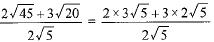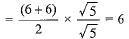Courses

# Test: RS Aggarwal: Real Numbers- 2

## 15 Questions MCQ Test Mathematics (Maths) Class 10 | Test: RS Aggarwal: Real Numbers- 2

Description
This mock test of Test: RS Aggarwal: Real Numbers- 2 for Class 10 helps you for every Class 10 entrance exam. This contains 15 Multiple Choice Questions for Class 10 Test: RS Aggarwal: Real Numbers- 2 (mcq) to study with solutions a complete question bank. The solved questions answers in this Test: RS Aggarwal: Real Numbers- 2 quiz give you a good mix of easy questions and tough questions. Class 10 students definitely take this Test: RS Aggarwal: Real Numbers- 2 exercise for a better result in the exam. You can find other Test: RS Aggarwal: Real Numbers- 2 extra questions, long questions & short questions for Class 10 on EduRev as well by searching above.
QUESTION: 1

### The decimal expansion of the rational number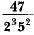will terminate after

Solution:

Multiply by 5 in both numerator and denominator
We get,
(47 × 5)/1000 = 0.235

QUESTION: 2

### Two alarm clocks ring their alarms at regular intervals of 50 seconds and 48 seconds. If they first beep together at 12 noon, at what time will they beep again for the first time?

Solution:

LCM of 50 and 48 = 1200
∴ 1200 sec = 20 min
Hence at 12.20 pm they will beep again for the first time.

QUESTION: 3

### The product of three consecutive integers is divisible by

Solution:

Let the three consecutive positive integers be n, n+1 and n+2.

Whenever a number is divided by 3, the remainder obtained is either 0,1 or 2.

Therefore, n=3p or 3p+1 or 3p+2, where p is some integer.

If n=3p, then n is divisible by 3.

If n=3p+1, then n+2=3p+1+2=3p+3=3(p+1) is divisible by 3.

If n=3p+2, then n+1=3p+2+1=3p+3=3(p+1) is divisible by 3.

So, we can say that one of the numbers among n,n+1 and n+2 is always divisible by 3 that is:

n(n+1)(n+2) is divisible by 3.

Similarly, whenever a number is divided by 2, the remainder obtained is either 0 or 1.

Therefore, n=2q or 2q+1, where q is some integer.

If n=2q, then n and n+2=2q+2=2(q+1) is divisible by 2.

If n=2q+1, then n+1=2q+1+1=2q+2=2(q+1) is divisible by 2.

So, we can say that one of the numbers among n, n+1 and n+2 is always divisible by 2.

Since, n(n+1)(n+2) is divisible by 2 and 3.

Hence, n(n+1)(n+2) is divisible by 6.

QUESTION: 4

If A = 2n + 13, B = n + 7, where n is a natural number, then HCF of A and B is:

Solution:

Taking different values of n we find that A and B are coprime
∴ HCF = 1

QUESTION: 5

The largest number which divides 615 and 963 leaving remainder 6 in each case is

Solution:

we have to  find the largest number which divide 615 and 963 leaving remainder 6 in each case.

so let us subtract 6 from 615 and 963

⇒ 615−6=609

⇒ 963−6=957

now lets find the HCF

⇒ prime factorisation of 609=29×3×3

prime factorisation of 957=29×3×11

now lets take out the common factors from both the cases

⇒ 29 and 3

x=29×3=87

∴ 87 is the number which will divide 615 and 963 leaving remainder 6 in each case.

QUESTION: 6

There are 576 boys and 448 girls in a school that are to be divided into equal sections of either boys or girls alone. The total number of sections thus formed are:

Solution:

HCF of 576 and 448 = 64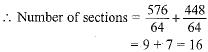QUESTION: 7

The largest number which divides 70 and 125 leaving remainders 5 and 8 respectively is

Solution:

The largest number by which x , y

divisible and gives the remainder a ,

and b is

the HCF of ( x - a ) and ( y - b)
According to the given problem ,

The largest number which divides

70 and 125 leaving remainders 5 and

8 respectively are

HCF of ( 70 - 5 ) = 65 and

( 125 - 8 ) = 117

65 = 5 × 13

117 = 3 × 3 × 13

HCF ( 65 , 117 ) = 13

Required number is 13.

QUESTION: 8

The HCF of 2472, 1284 and a third number N is 12. If their LCM is 23 x 32 x 5 x 103 x 107, then the number N is :

Solution: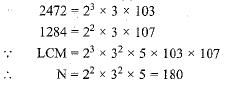QUESTION: 9

The product of a non zero rational and an irrational number is

Solution:
QUESTION: 10

Two natural numbers whose difference is 66 and the least common multiple is 360, are:

Solution:

Difference of 90 and 24 = 66
and LCM of 90 and 24 = 360
∴ Numbers are 90 and 24.

QUESTION: 11

The decimal expansion of the rational number 14587/1250 will terminate after

Solution:
QUESTION: 12

Which of the following rational numbers have a terminating decimal expansion?

Solution: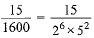The denominator 26 x 52 is of the form 2m x 5n, where m and n are non-negative integers. Hence, it is a terminating decimal expansion.

QUESTION: 13

If two positive integers a and b are written as a = x4 y2 and, b = x2 y3; a, b are prime numbers, then HCF (a, b) is

Solution:
QUESTION: 14

The LCM of two numbers is 1200. Which of the following cannot be their HCF?

Solution:

The LCM of two numbers = 1200.

By formula, product of two numbers =HCF x LCM

Let the two numbers be y,z

From the details given in question,

HCF x 1200 = y x z

The four options given in question are 600, 400, 500, and 200.

From the available options given, 500 cannot be the HCF since it cannot divide 1200

*Answer can only contain numeric values
QUESTION: 15

Evaluate: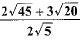Solution: